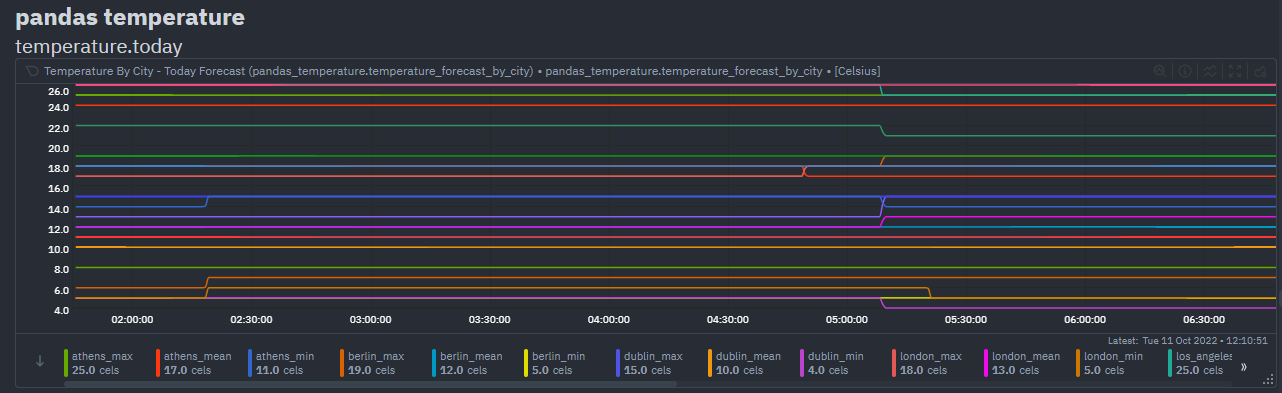# Ingest structured data (Pandas)Pandas is a de-facto standard in reading and processing most types of structured data in Python. If you have metrics appearing in a CSV, JSON, XML, HTML, or other supported format, either locally or via some HTTP endpoint, you can easily ingest and present those metrics in Netdata, by leveraging the Pandas collector.

The collector uses pandas to pull data and do pandas-based preprocessing, before feeding to Netdata.

## Requirements​

This collector depends on some Python (Python 3 only) packages that can usually be installed via `pip` or `pip3`.

``sudo pip install pandas requests``

Note: If you would like to use `pandas.read_sql` to query a database, you will need to install the below packages as well.

``sudo pip install 'sqlalchemy<2.0' psycopg2-binary``

## Configuration​

Below is an example configuration to query some json weather data from Open-Meteo, do some data wrangling on it and save in format as expected by Netdata.

``# example pulling some hourly temperature datatemperature:    name: "temperature"    update_every: 3    chart_configs:      - name: "temperature_by_city"        title: "Temperature By City"        family: "temperature.today"        context: "pandas.temperature"        type: "line"        units: "Celsius"        df_steps: >          pd.DataFrame.from_dict(            {city: requests.get(                f'https://api.open-meteo.com/v1/forecast?latitude={lat}&longitude={lng}&hourly=temperature_2m'                ).json()['hourly']['temperature_2m']             for (city,lat,lng)             in [                ('dublin', 53.3441, -6.2675),                ('athens', 37.9792, 23.7166),                ('london', 51.5002, -0.1262),                ('berlin', 52.5235, 13.4115),                ('paris', 48.8567, 2.3510),                ]            }            );                                                         # use dictionary comprehension to make multiple requests;          df.describe();                                               # get aggregate stats for each city;          df.transpose()[['mean', 'max', 'min']].reset_index();        # just take mean, min, max;          df.rename(columns={'index':'city'});                         # some column renaming;          df.pivot(columns='city').mean().to_frame().reset_index();    # force to be one row per city;          df.rename(columns={0:'degrees'});                            # some column renaming;          pd.concat([df, df['city']+'_'+df['level_0']], axis=1);       # add new column combining city and summary measurement label;          df.rename(columns={0:'measurement'});                        # some column renaming;          df[['measurement', 'degrees']].set_index('measurement');     # just take two columns we want;          df.sort_index();                                             # sort by city name;          df.transpose();                                              # transpose so its just one wide row;``

`chart_configs` is a list of dictionary objects where each one defines the sequence of `df_steps` to be run using `pandas`, and the `name`, `title` etc to define the CHART variables that will control how the results will look in netdata.

The example configuration above would result in a `data` dictionary like the below being collected by Netdata at each time step. They keys in this dictionary will be the "dimensions" of the chart.

``{'athens_max': 26.2, 'athens_mean': 19.45952380952381, 'athens_min': 12.2, 'berlin_max': 17.4, 'berlin_mean': 10.764285714285714, 'berlin_min': 5.7, 'dublin_max': 15.3, 'dublin_mean': 12.008928571428571, 'dublin_min': 6.6, 'london_max': 18.9, 'london_mean': 12.510714285714286, 'london_min': 5.2, 'paris_max': 19.4, 'paris_mean': 12.054166666666665, 'paris_min': 4.8}``

Which, given the above configuration would end up as a chart like below in Netdata.• Each line in `df_steps` must return a pandas DataFrame object (`df`) at each step.
• You can use this colab notebook to mock up and work on your `df_steps` iteratively before adding them to your config.
• This collector is expecting one row in the final pandas DataFrame. It is that first row that will be taken as the most recent values for each dimension on each chart using (`df.to_dict(orient='records')`). See pd.to_dict().